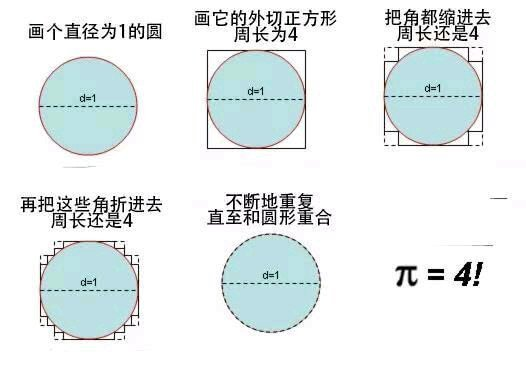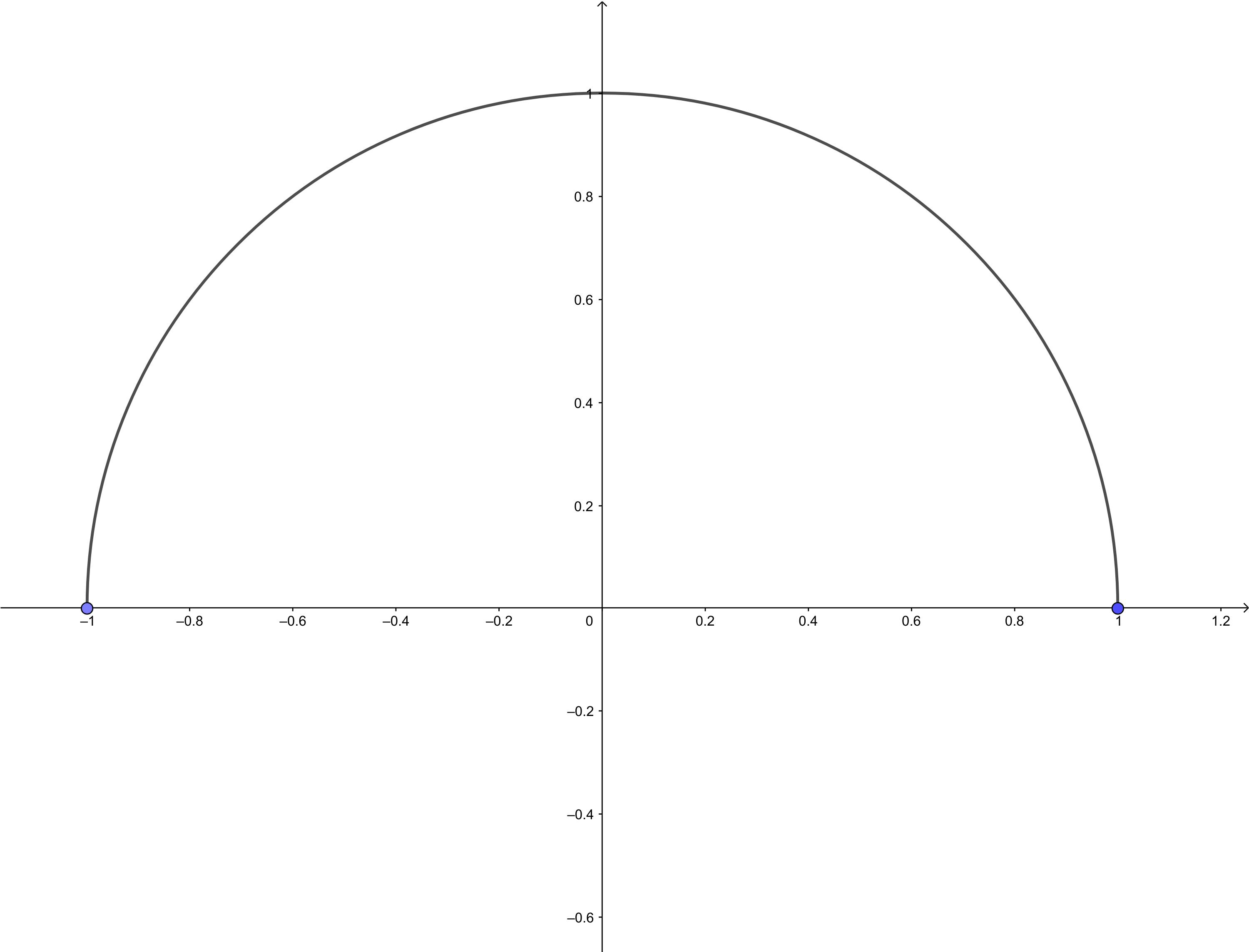# 为什么是错误的?

## 一个图形怎样才是圆形?1. $g(x) = f(x)$
2. 函数曲线的长度为 $\pi$ (即等于该半圆长度)

• 不断的重复后正方形最终折叠成一个半圆

事实上这种想法用数学语言解释其实就是认为, 不断重复后的正方形的表达其实是 (此时正方形必须斜放, 不然不会有函数表达式, 但是这只是为了方便, 并不影响最终结果)
$$\lim_{n\to\infty} g_n(x)$$
并且有
$$\lim_{n\to\infty} g_n(x)=f(x)$$
按照指标 1 自然可以认为最终就是得到一个半圆.

• 不断的重复后最终并不是半圆

我们让 $l(g_n(x))$ 表示 $g_n(x)$ 函数曲线的长度 . 那么有
$$\lim_{n\to\infty}l(g_n(x))=4\not-\pi$$
根据指标 1 就可以认为最终其实不是圆.

## 那为什么不能证明 π = 4 ?

$$\lim_{x\to\infty}l(g_n(x))\not=l(\lim_{x\to\infty}g_n(x))$$